## Learning Objectives

Use the electric (E) vector of light to describe the behavior of plane polarized light.

## Prior Knowledge and Skills

3.4 Electric and Magnetic Components of Light

## Key Terms

Electric Vector (E)

## Guided Inquiry

Polarized Light (note: only need to watch linear polarization, to 6:00, but feel free to watch the whole video!)

https://youtu.be/gP751qpm4n4

Polaroid sunglasses are familiar to most of us. They have a special ability to cut the glare of light reflected from water or glass (see Figure 3.5.1). Polaroids have this ability because of a wave characteristic of light called polarization. What is polarization? How is it produced? What are some of its uses? The answers to these questions are related to the wave character of light.Figure 3.5.1. These two photographs of a river show the effect of a polarizing filter in reducing glare in light reflected from the surface of water. Part (b) of this Figure was taken with a polarizing filter and part (a) was not. As a result, the reflection of clouds and sky observed in part (a) is not observed in part (b). Polarizing sunglasses are particularly useful on snow and water. (credit: Amithshs, Wikimedia Commons)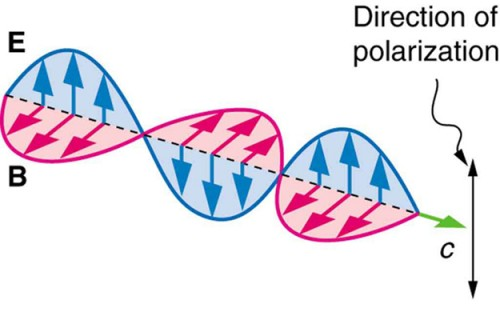Figure 3.5.2. An EM wave, such as light, is a transverse wave. The electric and magnetic fields are perpendicular to the direction of propagation.

Light is one type of electromagnetic (EM) wave. As noted earlier, EM waves are transverse waves consisting of varying electric and magnetic fields that oscillate perpendicular to the direction of propagation (see Figure 3.5.2). There are specific directions for the oscillations of the electric and magnetic fields. Polarization is the attribute that a wave’s oscillations have a definite direction relative to the direction of propagation of the wave. (This is not the same type of polarization as that discussed for the separation of charges.) Waves having such a direction are said to be polarized. For an EM wave, we define the direction of polarization to be the direction parallel to the electric field. Thus we can think of the electric field arrows as showing the direction of polarization, as in Figure 3.5.2.

To examine this further, consider the transverse waves in the ropes shown in Figure 3.5.3. The oscillations in one rope are in a vertical plane and are said to be vertically polarized. Those in the other rope are in a horizontal plane and are horizontally polarized. If a vertical slit is placed on the first rope, the waves pass through. However, a vertical slit blocks the horizontally polarized waves. For EM waves, the direction of the electric field is analogous to the disturbances on the ropes.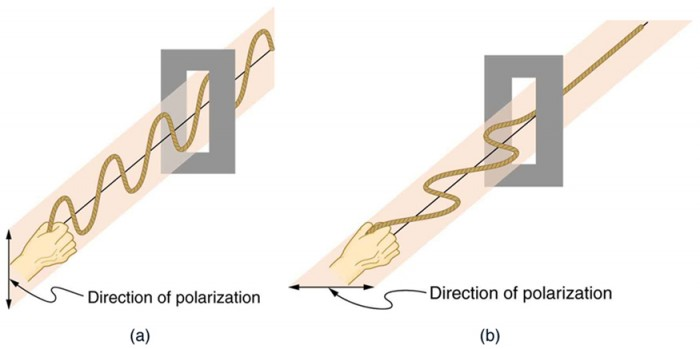Figure 3.5.3. The transverse oscillations in one rope are in a vertical plane, and those in the other rope are in a horizontal plane. The first is said to be vertically polarized, and the other is said to be horizontally polarized. Vertical slits pass vertically polarized waves and block horizontally polarized waves.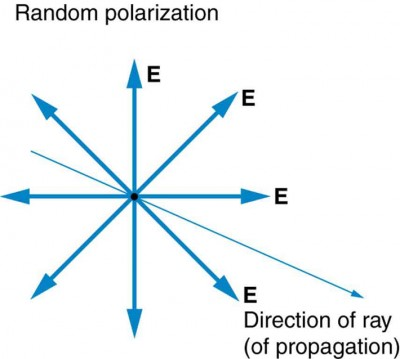Figure 3.5.4. The slender arrow represents a ray of unpolarized light. The bold arrows represent the direction of polarization of the individual waves composing the ray. Since the light is unpolarized, the arrows point in all directions.

The Sun and many other light sources produce waves that are randomly polarized (see Figure 3.5.4). Such light is said to be unpolarized because it is composed of many waves with all possible directions of polarization. Polaroid materials, invented by the founder of Polaroid Corporation, Edwin Land, act as a polarizing slit for light, allowing only polarization in one direction to pass through. Polarizing filters are composed of long molecules aligned in one direction. Thinking of the molecules as many slits, analogous to those for the oscillating ropes, we can understand why only light with a specific polarization can get through. The axis of a polarizing filter is the direction along which the filter passes the electric field of an EM wave (see Figure 3.5.5).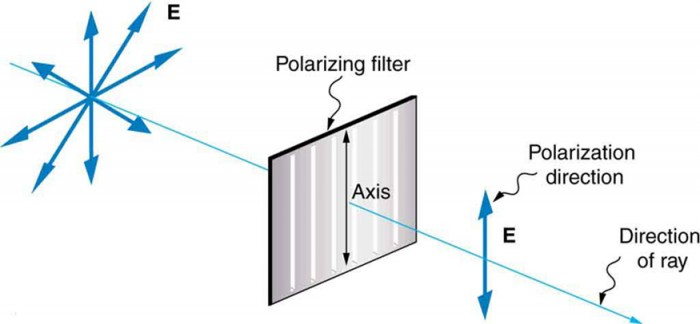Figure 3.5.5. A polarizing filter has a polarization axis that acts as a slit passing through electric fields parallel to its direction. The direction of polarization of an EM wave is defined to be the direction of its electric field.

Figure 3.5.6 shows the effect of two polarizing filters on originally unpolarized light. The first filter polarizes the light along its axis. When the axes of the first and second filters are aligned (parallel), then all of the polarized light passed by the first filter is also passed by the second. If the second polarizing filter is rotated, only the component of the light parallel to the second filter’s axis is passed. When the axes are perpendicular, no light is passed by the second.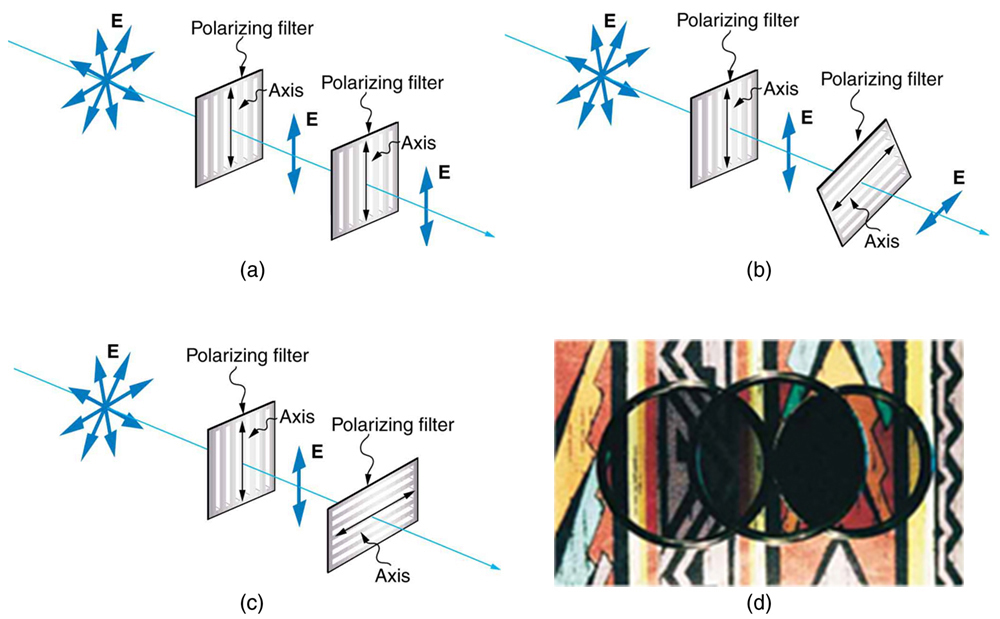Figure 3.5.6. The effect of rotating two polarizing filters, where the first polarizes the light. (a) All of the polarized light is passed by the second polarizing filter, because its axis is parallel to the first. (b) As the second is rotated, only part of the light is passed. (c) When the second is perpendicular to the first, no light is passed. (d) In this photograph, a polarizing filter is placed above two others. Its axis is perpendicular to the filter on the right (dark area) and parallel to the filter on the left (lighter area). (credit: P.P. Urone)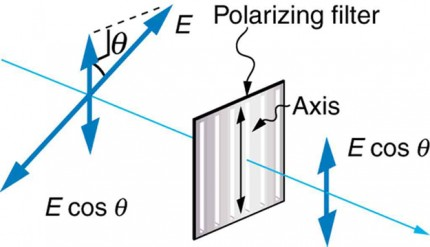Figure 3.5.7. A polarizing filter transmits only the component of the wave parallel to its axis, , reducing the intensity of any light not polarized parallel to its axis.

Only the component of the EM wave parallel to the axis of a filter is passed. Let us call the angle between the direction of polarization and the axis of a filter θ. If the electric field has an amplitude E, then the transmitted part of the wave has an amplitude E cos θ (see Figure 3.5.7). Since the intensity of a wave is proportional to its amplitude squared, the intensity I of the transmitted wave is related to the incident wave by I = I0 cos2 θ, where I0 is the intensity of the polarized wave before passing through the filter. (The above equation is known as Malus’s law.)

3.5.1. Is the polarization direction of light parallel to a) the electric vector; b) the magnetic vector;  or c) the direction of light propagation?

3.5.2. Is all light polarized?

3.5.3. How can you check the polarization direction of one polarizer by using a second polarizer?

Concept Check 3.5.1

Is laser light polarized or unpolarized? What is an important implication for this if you were trying to analyze an anisotropic mineral sample with a laser?

## Summary

Want more information about polarization of light? See the OpenStax / Lumen learning physics textbook chapters referenced below.

## References

Kevin Claytor. Polarized Light. (Nov 9, 2013) https://youtu.be/gP751qpm4n4

OpenStax, College Physics. OpenStax CNX. Mar 4, 2019 http://cnx.org/contents/031da8d3-b525-429c-80cf-6c8ed997733a@14.48.

MIT OpenCourseWare. Laser fundamentals I: Polarization of laser light | MIT Video Demonstrations in Lasers and Optics. (June 15, 2012)  https://youtu.be/mjwQTL6G8Fs